Select Page

# Maths Three Dimensional Geometry CBSE 12 Science MCQ in English Solutions

Maths Three Dimensional Geometry CBSE 12 Science MCQ in English Solutions to enable students to get Solutions in a narrative video format for the specific question.

Expert Teacher provides Maths Three Dimensional Geometry CBSE 12 Science MCQ English Solutions. This video solution will be useful for students to understand how to write an answer in exam in order to score more marks. This teacher uses a narrative style for a question from Three Dimensional Geometry not only to explain the proper method of answering question, but deriving right answer too.

Please find the question below and view the Solution in a narrative video format.

Question:

Solution Video in English:

## Similar Questions from CBSE, 12th Science, Maths, Three Dimensional Geometry

Question 1 : Find the vector equation of a plane which is at a distance of 5 units from the origin and its normal vector is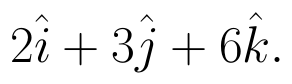(View Answer Video)

Question 2 : A plane makes intercepts -6, 3, 4 respectively on the co-ordinte axes. Find the length of the perpendicular from the origin on it. (View Answer Video)

Question 3 : Find the equation of plane passing through the line of intersection of the planes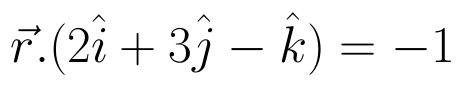and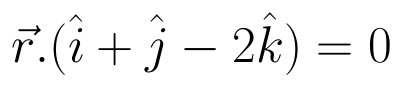and passing through the point (3, -2, -1). Also, find the angle between the two given planes. (View Answer Video)

Question 4 : A line passes through the point with position vector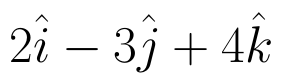and makes angles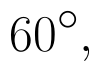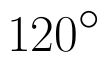and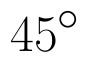with x, y and z-axis respectively. Find the equation of the line in the Cartesian form. (View Answer Video)

Question 5 : The equation of a line AB is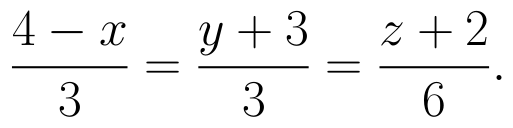Write the direction cosines of a line parallel to the above line. (View Answer Video)

### Determinant

Question 1 : The following system of equations has x + 3y + 3z = 2, x + 4y + 3z = 1, x + 3y + 4z = 2,

Question 2 : If area of a triangle is 35 sq unit with vertices (2, -6), (5, 4) and (k, 4), then k is, (View Answer Video)

Question 3 : Evaluate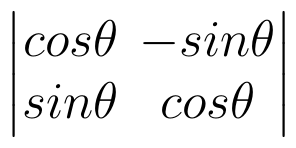. (View Answer Video)

Question 4 : Evaluate the determinant: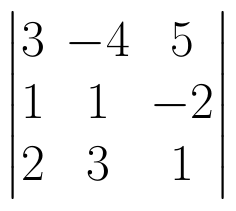. (View Answer Video)

Question 5 : Find the values of x, if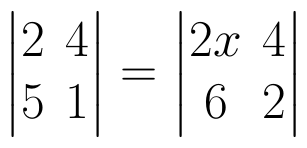. (View Answer Video)

### Vector Algebra

Question 1 : Find the unit vector in the direction of vector PQ, where P and Q are the points (1, 2, 3) and (4, 5, 6) respectively. (View Answer Video)

Question 2 :  Write a unit vector in the direction of the sum of vectors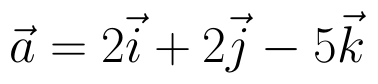and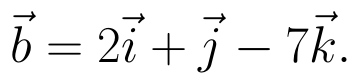(View Answer Video)

Question 3 :  If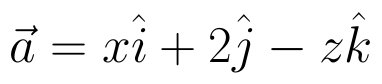and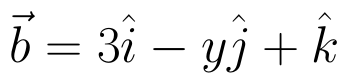are two equal vectors, then write the value of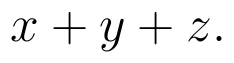(View Answer Video)

Question 4 :  Write the value of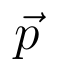for which the vectors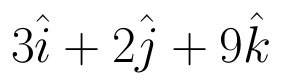and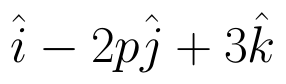are parallel vectors.  (View Answer Video)

Question 5 : Find the angle between two vectors a and b with magnitudes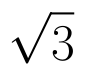and 2 respectively, having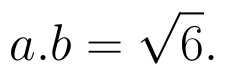(View Answer Video)

### Differential Equations

Question 1 : Form the differential equation of the family of circles in the second quadrant and touching the co-ordinate axes. (View Answer Video)

Question 2 : Write the differential equation representing the family of curves y = mx, where m is an arbitrary constant. (View Answer Video)

Question 3 : Find the particular solution of the differential equation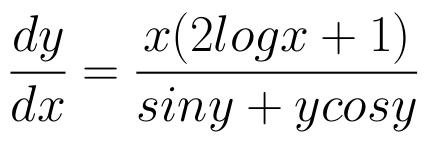given that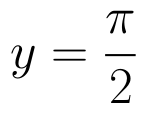where x = 1. (View Answer Video)

Question 4 : If m and n are the order and degree, respectively of the differential equation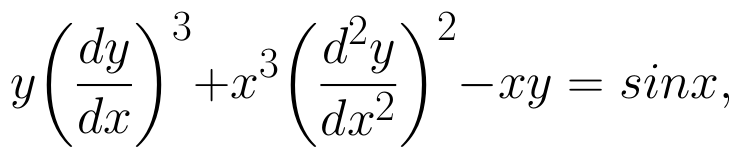then write value of m+n. (View Answer Video)

Question 5 : Find the solution of the differential equation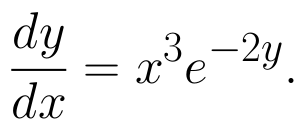(View Answer Video)